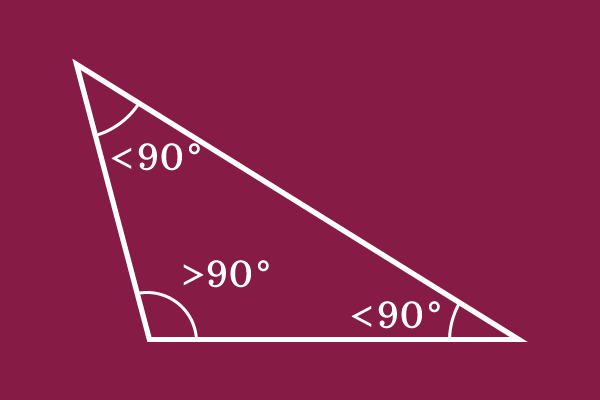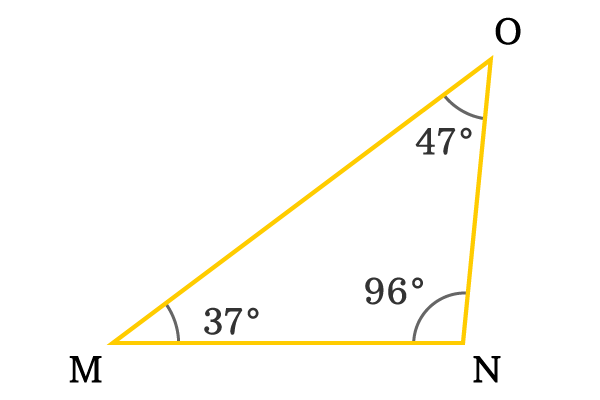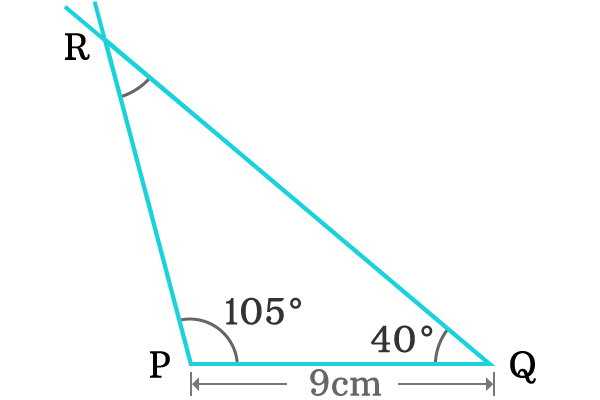# Obtuse Angled Triangle## Definition

A triangle whose one of three interior angles is an obtuse angle, is called an obtuse angled triangle.

A triangle can have an obtuse angle as one of its three interior angles and the remaining angles are acute angles. Due to having obtuse angle as interior angle of the triangle, the triangle is named as obtuse angled triangle.

### Properties

$\Delta MNO$ is a fundamental example to obtuse angled triangle to study its properties.###### First Interior Angle

The $\angle OMN$ is formed by the sides $\overline{OM}$ and $\overline{MN}$ at vertex $M$.

$\angle OMN = 37^\circ$

The interior angle ($\angle OMN$) is less than $90^\circ$. So, it is an acute angle.

###### Second Interior Angle

The $\angle MNO$ is formed by the sides $\overline{MN}$ and $\overline{NO}$ at vertex $N$.

$\angle MNO = 96^\circ$

The interior angle ($\angle MNO$) is greater than $90^\circ$. So, it is an obtuse angle.

###### Third Interior Angle

The $\angle NOM$ is formed by the sides $\overline{NO}$ and $\overline{OM}$ at vertex $O$.

$\angle NOM = 47^\circ$

The interior angle ($\angle NOM$) is less than $90^\circ$. So, it is an acute angle.

In $\Delta MNO$, two interior angles are acute angles but one interior angle is obtuse angle. Hence, the triangle $MNO$ is called as an obtuse angled triangle.

### Construction

An obtuse angled triangle can be constructed geometrically by using geometric tools to understand the properties practically.1. Take ruler and draw horizontal line of any length. Here, $9$ Centimetres horizontal line is drawn and its endpoints are called as point $P$ and point $Q$.
2. Take protractor, coincide its centre with point $P$ and also coincide its right side base line with line segment $\overline{PQ}$. After that, mark a point at any obtuse angle (For example $105^\circ$) by considering bottom scale.
3. Use protractor again, coincide point $Q$ with its centre and also coincide its left side baseline with line segment $\overline{PQ}$. Identify any acute angle by considering top scale. In this case, a point is marked at $40^\circ$ angle.
4. Draw a line from point $P$ through $105^\circ$ angle marked point and also draw a line from point $Q$ through $40^\circ$ angle marked point. The two extended lines intersect at a point $R$.

An obtuse angled triangle ($\Delta PQR$) is constructed successfully by this geometrical procedure. The points $P$, $Q$ and $R$ are vertices of the triangle. The line segments $\overline{PQ}$, $\overline{QR}$ and $\overline{RP}$ are sides of the triangle.

1. The interior angle ($\angle RPQ$) is known and it is $105^\circ$. This interior angle is greater than $90^\circ$ and it is known as obtuse angle. Due to formation of triangle by an obtuse angle, the $\Delta PQR$ is called as an obtuse angled triangle.
2. The second interior angle ($\angle PQR$) is $40^\circ$ and it is an acute angle.
3. The third interior angle ($\angle QRP$) is unknown but it’s not an issue and measure it by using a protractor. It is measured as $35^\circ$ exactly.
Latest Math Topics
Latest Math Problems
Email subscription
Math Doubts is a best place to learn mathematics and from basics to advanced scientific level for students, teachers and researchers. Know more